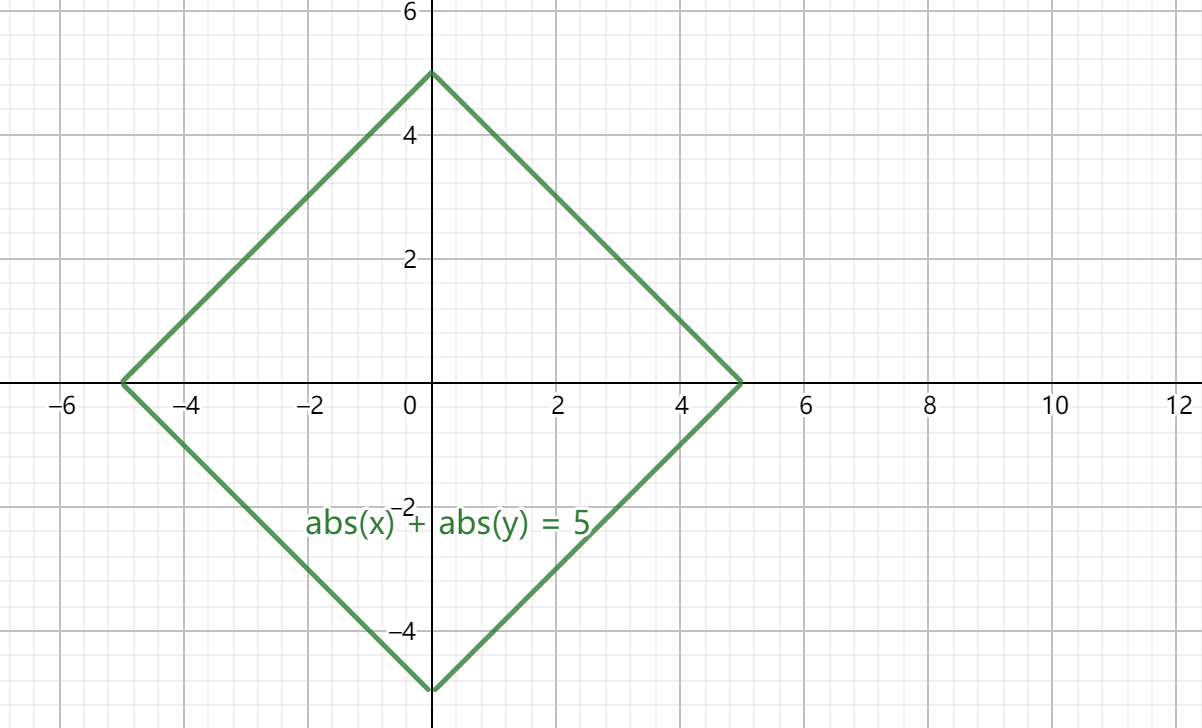true
Update at 2021.12.14 B题 更新一份Hack数据构造代码

## 我永远喜欢樱岛麻衣（思维）#

$(x_1,y_1),(x_2,y_2)$ 之间的曼哈顿距离为 $d=|x_1-x_2|+|y_1+y_2|$ ，即两点各方向上坐标之差的绝对值的和。## lq的简单题（思维，map）#

Hack思路

32位整值溢出可以理解为在模 $2^{32}$ 意义下同余，那么代码实际求值会包括一部分满足下面式子的数对

$$n\times(2\times avg - a_i - a_j)\equiv 0 \pmod{2^{32}}$$

  1 2 3 4 5 6 7 8 9 10 11 12 13 14 15 16 17 18 19 20 21 22 23 24 25 26 27 28 29 30 31 32 33  #include using namespace std; mt19937 rng(chrono::steady_clock::now().time_since_epoch().count()); int randint(int l, int r) { return uniform_int_distribution(l, r)(rng); } int main() { ios_base::sync_with_stdio(false), cin.tie(nullptr), cout.tie(nullptr); vector out; int x, y, s, n = 1 << 16; // s = 2 * avg do { x = randint(1, (int) 4e5), y = randint(1, (int) 4e5); s = x + y + n; } while (s > (int) 8e5 || n + x > (int) 4e5 || n + y > (int) 4e5); out.emplace_back(x), out.emplace_back(n + y); out.emplace_back(y), out.emplace_back(n + x); for (int t; (int) out.size() != n; ) { do { t = randint(1, min((int) 4e5, s)); } while (s - t > (int) 4e5 || s - t < 1); out.emplace_back(t), out.emplace_back(s - t); } random_shuffle(out.begin(), out.end()); cout << "1\n" << n << '\n'; for (int x : out) { assert(x >= 1 && x <= (int) 4e5); cout << x << ' '; } return 0; } 

## 哥德巴赫猜想（数论，玄学/预处理）#

（注意使用 $O(\sqrt n)$ 的试除法判别素性并记录，题目卡了 $O(n)$ 的试除法）。

PS：如果你在本地测试过（或着直接莽一发）或着尝试打表（打表指在本地算完结果，再复制到代码里的行为），应该来说是会发现看似暴力的复杂度其实可以接受。

（数论部分的内容会在寒假以直播的形式讲，其中会涉及这部分筛法，若条件允许还会讲一种十分优秀的素性判别法，其时间复杂度约为 $O(k\log^3 n)$ ）

## 数学家uli（思维/差分）#

### 1. 差分（出题人本意）#

 1 2 3  for (int x = A; x <= B; x++) for (int y = B; y <= C; y++) cnt[x + y]++; 

### 2. 思维（验题过程中发现的更好的写法）#

$x+y=k\Rightarrow x=k-y$，又有 $A\leq x\leq B\leq y\leq C$ ，可知 $x\in [A,B]\bigcap[k-C,k-B]$ 。

## 攻城掠地（二分/DP）#

### 1. 二分#

• 如果中途无法使左侧全1，则返回false

• 如果左侧必须要m1，则必须首先拓展左侧

• 反之直接先拓展右侧（使之更远）

• 最终位置如果超出或恰好为数组末尾则m为一个可行解，返回true

### 2. DP#

• 第一个1可以直接处理
• 第 $i$ 和第 $i-1$ 个1，考虑转移，一共四种状态。无论哪种状态，影响的只有二者之间的除去第一步之后0的数目，这部分除以 $2$ 向上取整的结果 和 第 $i-1$ 个1在该状态下的dp值取最大值（取最大值是因为必须保证第 $i-1$ 个1前全为1）即为第 $i$ 个1在该状态下的dp值。(ps：注意负数的处理)

## 凉心出题人（签到，DP）#

 1  dp[i] = dp[i - 1] + dp[i - 2]